Courses

# Failure Theories and Concrete Plasticity (Part - 1) Civil Engineering (CE) Notes | EduRev

## Civil Engineering (CE) : Failure Theories and Concrete Plasticity (Part - 1) Civil Engineering (CE) Notes | EduRev

The document Failure Theories and Concrete Plasticity (Part - 1) Civil Engineering (CE) Notes | EduRev is a part of Civil Engineering (CE) category.
All you need of Civil Engineering (CE) at this link: Civil Engineering (CE)

Failure Theories and Concrete Plasticity
→ Failure of concrete
o Concrete is a brittle material which fails through brittle cleavage (splitting) at the interfaces and in mortar except for high triaxial compression where shear slippage occurs resulting in a ductile behavior. Failure occurs by tensile splitting with the fractured surface orthogonal to the direction of the maximum tensile stress or strain.

→ Prediction of multiaxial behavior
o In general the material properties are known from simple tests such as uniaxial loadings giving  f'c and ft . Prediction involves strength calculation in multiaxial situations given the data from the uniaxial tests.
o In the field of concrete research attempts have been made to apply some of the classical failure theories to concrete. These theories were altered to overcome some disadvantages or otherwise improve their agreement with the phenomenological behavior of concrete. New failure theories were therefore formed.

→ Principal stresses: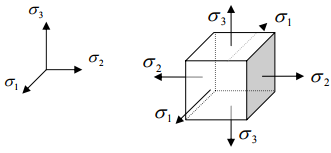→ Some classical failure theories
o Maximum principal stress theory
o Maximum principal strain theory
o Maximum shear stress theory
o Internal friction theory
o Maximum strain energy theory
o Distortion energy theory
o Fracture mechanics based theories – stress intensity, toughness – fracture energy release.

→ These introduce either limitations or contradictions when applied to concrete. Modifications to concrete have resulted:
o Internal friction-maximum stress theory
o Octahedral shear-normal stress theory
o Newman’s two-part criterion
o Local deformation theories, etc.

→ Extensive research has been conducted to develop better theories: Elastic-plastic, plastic-fracturing, endochronic, bounding surface etc. approaches.

→ Maximum principal stress theory (elastic behavior)
o σ1  > σ2 > σ3
o Failure occurs when: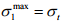(Tensile strength) max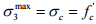(Compressive strength)
→ It does not reflect splitting nature of failure.

→ Maximum principal strain theory (elastic behavior)
o Failure occurs when: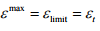→ Maximum shear stress theory
o σ1  > σ2 > σ3
o Failure occurs when:
σ1 − σ3 + λ (σ13 ) = 2σs
where σ1 −σ3 = shear stress,
λ (σ13 ) = portion of the volumetric stress,
σs = a critical shear stress value (e.g. under pure shear)

o For metals, λ ≅ 0 . For brittle materials, λ ≠ 0 .
o For λ = 0 , the failure criterion becomes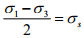The theory gives equal uniaxial tensile and compressive strengths. It is also independent of intermediate stress σ2 . (pressure sensitivity)

→ Internal friction theory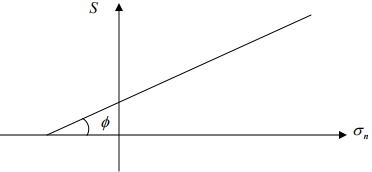o Consider the effect of normal stress on shear strength:
S = K − tan φσn
where S = shear strength,
K = cohesive strength,
φ = angle of internal friction, and
σn = normal stress.

o Compression increases S and tension decreases S .

Mohr’s theory (generalization of internal friction theory)

o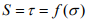o σ2 has no effect on strength.
o f (σ ) is the envelop of all the circles corresponding to the various states of stress at which failure takes place.

Octahedral shear and normal stress theory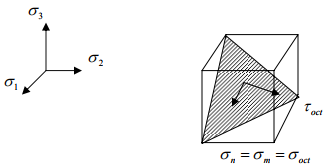o σ1 > σ> σ3
Failure occurs when the octahedral stress exceeds a limiting value.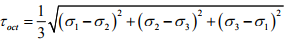In uniaxial tension and compression,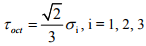o The failure criterion provided by octahedral shear stress theory: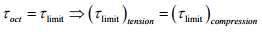→ This gives the same ultimate strength for uniaxial tension and compression.→ It is not valid for concrete.
→ Inclusion of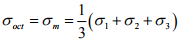improves the prediction.

o Bresler, Pister tests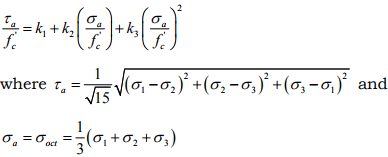→ Invariant formulation
o A failure criterion should be based upon an invariant function of the state of stress, i.e., independent of the choice of the coordinate systems.
o Stress invariants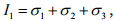(more suitable for applying to concrete)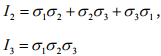where σ1,σ23 are principal stresses.

General stress state representation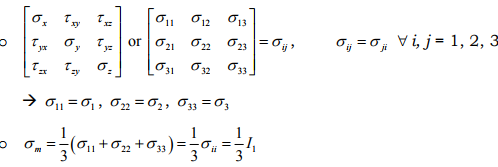o Average normal stress: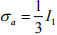o Average shear stress: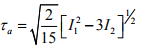Offer running on EduRev: Apply code STAYHOME200 to get INR 200 off on our premium plan EduRev Infinity!

,

,

,

,

,

,

,

,

,

,

,

,

,

,

,

,

,

,

,

,

,

;